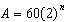Name:    Exponential growth & Decay

Multiple Choice
Identify the choice that best completes the statement or answers the question.

1.

A colony of ants has an initial population of 200 and doubles every day. A function that can be used to model the ant population, p, after t days is
 a.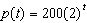c.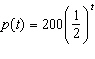b.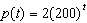d.2.

A bacteria colony initially has 4000 cells and triples every week. A function that can be used to model the population, p, of the colony after t days is
 a.c.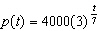b.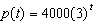d.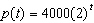3.

A series of shapes are constructed from blocks. The first shape is made from 3 blocks. Each successive shape is made using twice as many blocks as the previous shape. The total number of blocks required for the first 4 shapes is
 a. 24 blocks c. 42 blocks b. 36 blocks d. 45 blocks

4.

Mohamed purchased a car for \$30 000. It depreciates by 25% of its current value every year. How much will the car be worth 3 years after it is purchased?
 a. \$12 656.25 c. \$16 875 b. \$71 111.11 d. \$22 500

5.

The equation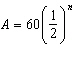can also be written as
 a.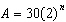c.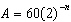b.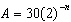d.